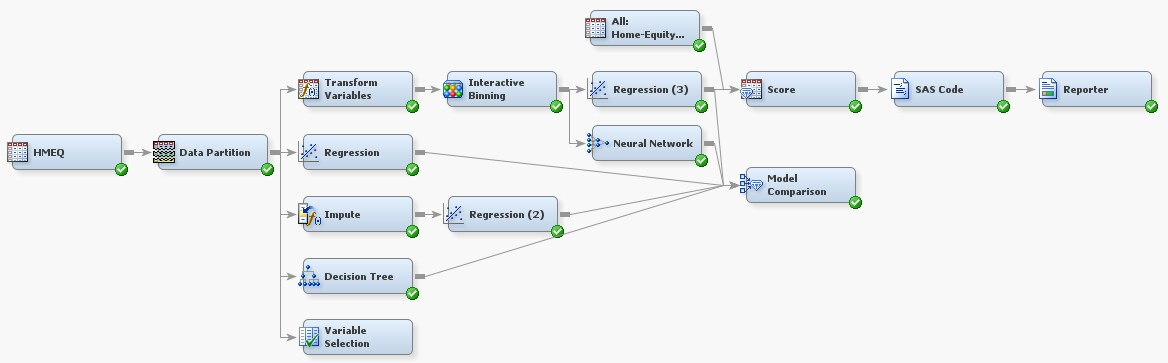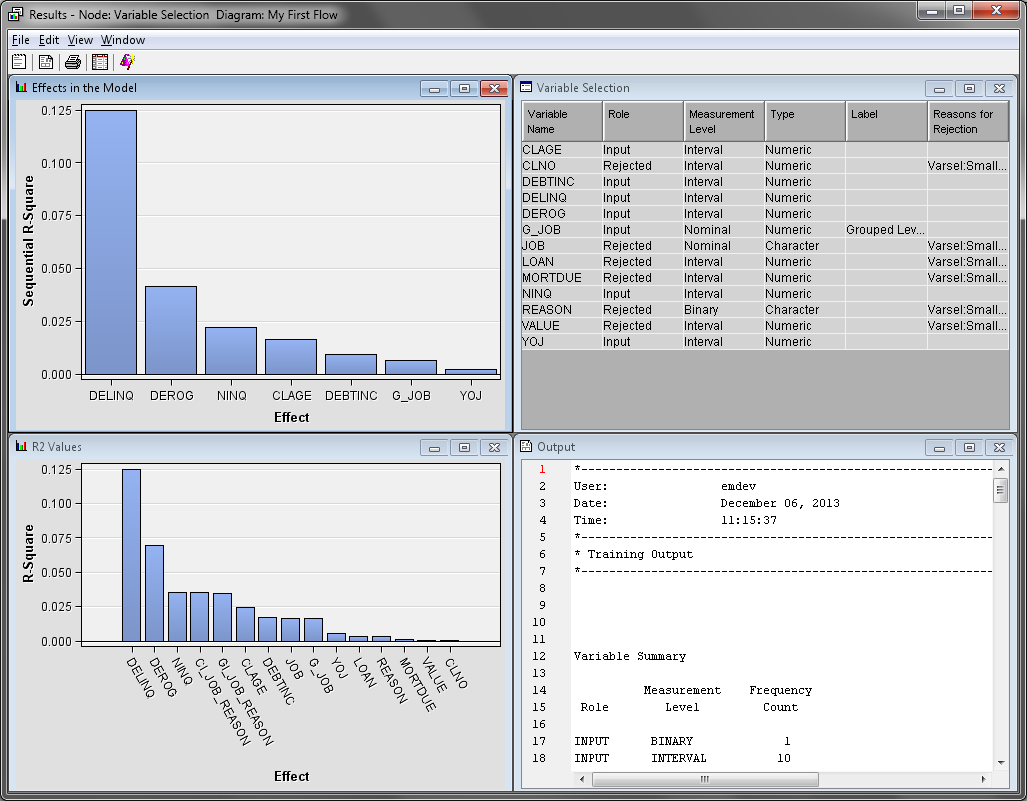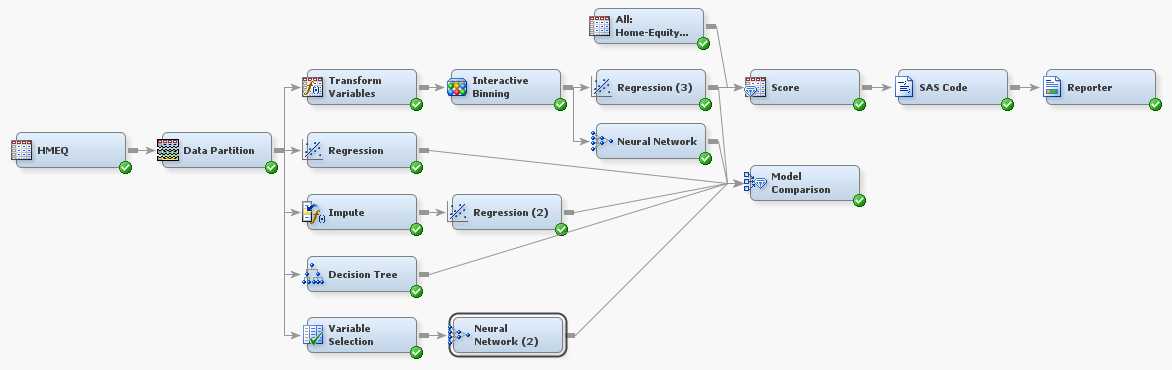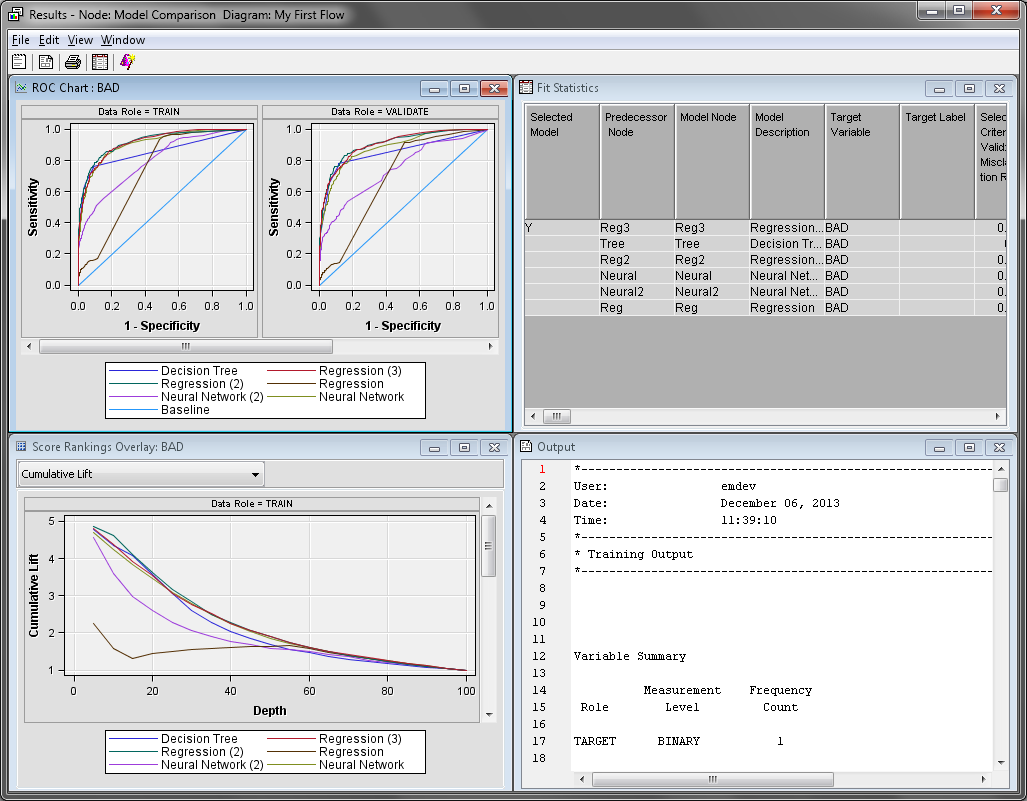Using the Variable Selection Node

Selecting Variables Using the R-Square Criterion

On the Explore tab, drag a Variable Selection node to your diagram workspace. Connect the Data Partition node to the Variable Selection node.Set the value of the Max Missing Percentage property to `10`. This eliminates variables that have more than 10% of their values missing.
Set the value of the Target Model property to R-Square. This indicates that the r-square criterion is used to evaluate and select variables. Notice that the Chi-Square Options properties subgroup is now unavailable. Use the default values of the properties in the R-Square Options subgroup.
The R-Square criterion uses a goodness-of-fit criterion to evaluate variables. It uses a stepwise method of selecting variables that stops when the improvement in the r-square value is less than 0.0005. By default, this method rejects variables whose contribution to the r-square value is less than 0.005.
The following three-step process is done when you apply the R-Square criterion to a binary target. When the target is non-binary, only the first two steps are performed.
1. SAS Enterprise Miner computes the squared correlation for each variable with the target and then assigns the rejected role to those variables that have a value less than Minimum R-Square value.
2. SAS Enterprise Miner evaluates the remaining variables with a forward stepwise r-square regression. Variables that have a stepwise r-square improvement less than the Stop R-Square value are rejected.
3. SAS Enterprise Miner performs a logistic regression with the predicted values that are output from the forward stepwise regression used as the independent input variable.
Right-click the Variable Selection node and click Run. In the Confirmation window, click Yes. Click Results in the Run Status window.In the Variable Selection window, notice that CLAGE, DEBTINC, DELINQ, DEROG, G_JOB, NINQ, and YOJ have their Role set to Input. This indicates that they were the variables selected by the node for inclusion in the preceding neural network model.
Close the Results window.

Creating and Evaluating a Neural Network Model

On the Model tab, drag a Neural Network node to your diagram workspace. Connect the Variable Selection node to the Neural Network (2) node. Connect the Neural Network (2) node to the Model Comparison node.It is highly recommended that you perform some type of variable selection before building neural network models. Neural network models are very flexible, but they are also very computationally intense. Failure to reduce the number of input variables can result in the following:
• an overfit model that does not perform well in practice
• a tremendous increase in the computational time that is necessary to fit a model
• computational difficulties in obtaining good parameter estimates
As in the previous chapter, use the default settings for the Neural Network (2) node. Right-click the Model Comparison node and click Run. In the Confirmation window, click Yes. Click Results in the Run Status window.Notice that the Neural Network (2) model, while significantly better than the initial Regression model, is considered worse than the other models. Close the Results window.
As an exercise, consider adjusting the R-Square Options or setting the Target Model property to Chi-Square.